Question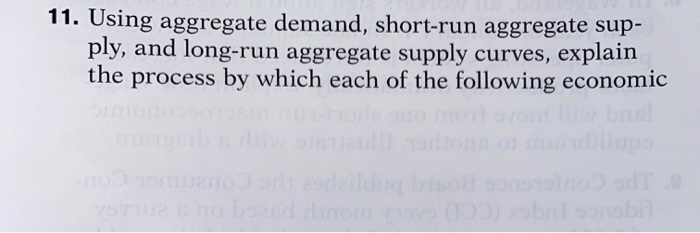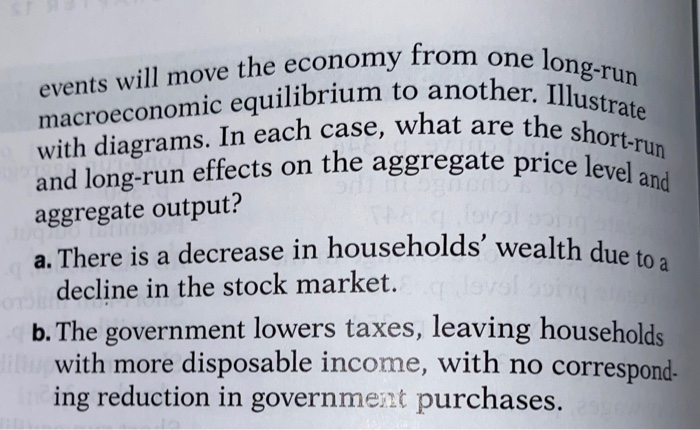a. A decrease in households’ wealth will reduce consumer spending. Beginning at long-run macroeconomic equilibrium, E1 in the diagram, the aggregate demand curve will shift from AD1 to AD2. In the short run, nominal wages are sticky, and the economy will be in short-run macroeconomics equilibrium at point E2. The aggregate price level will be lower than at E1, and aggregate output will be lower than the potential output. The end result is people will have less money to spend.

b. An increase in disposable income will increase consumer spending; at any given aggregate price level, the aggregate demand curve will shift to the right, in the long run at EQ1.  The economy will spend more and the aggregate output will be higher than potential output. In the short-run, nominal wages are sticky, and the economy will be in the short-run at EQ2.

#### Earn Coins

Coins can be redeemed for fabulous gifts.

Similar Homework Help Questions
• ### Using aggregate demand (AD), short-run aggregate supply (SRAS) and long-run aggregate supply (LRAS) curves

Question 1: AD-SRAS-LRAS Model Using aggregate demand (AD), short-run aggregate supply (SRAS) and long-run aggregate supply (LRAS) curves, graphically illustrate the effect of an increase in the money supply on output and prices in the short and long run. Assume that the economy is initially in long run equilibrium at the potential output level and prices are fixed in the short-run. In your graph, label "A" for the initial equilibrium, "B' for the short-run equilibrium, and "C" for the long-run equilibrium.

• ### The graph below depicts the aggregate demand, Irrun aggregate supply, and short-run aggregate supply curves for...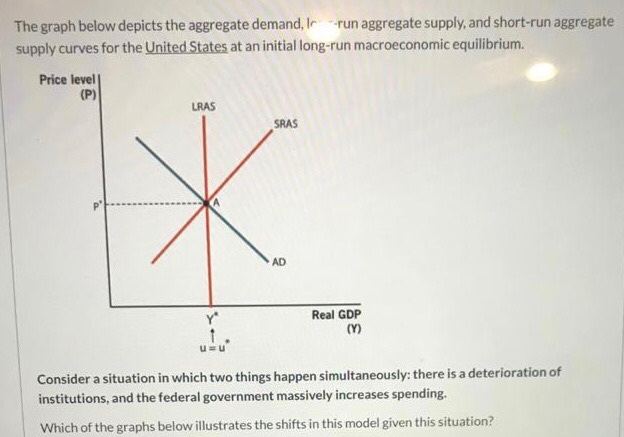The graph below depicts the aggregate demand, Irrun aggregate supply, and short-run aggregate supply curves for the United States at an initial long-run macroeconomic equilibrium Price level] (P) LRAS SRAS Real GDP Consider a situation in which two things happen simultaneously: there is a deterioration of institutions, and the federal government massively increases spending. Which of the graphs below illustrates the shifts in this model given this situation? Price level Price level (P) (P) URAS LRAS, LRAS SRAS SRAS SRAS...

• ### Using the aggregate demand (AD), the short-run aggregate supply (SRAS), and the long-run aggregate supply (LRAS)...

Using the aggregate demand (AD), the short-run aggregate supply (SRAS), and the long-run aggregate supply (LRAS) curves, briefly explain how an open market purchase will affect the equilibrium price level (P) and real output (Y) in the short run. Assume the economy is initially in a recession?

• ### Below, you are provided with the aggregate demand, short-run aggregate supply, and long-run aggregate supply curves....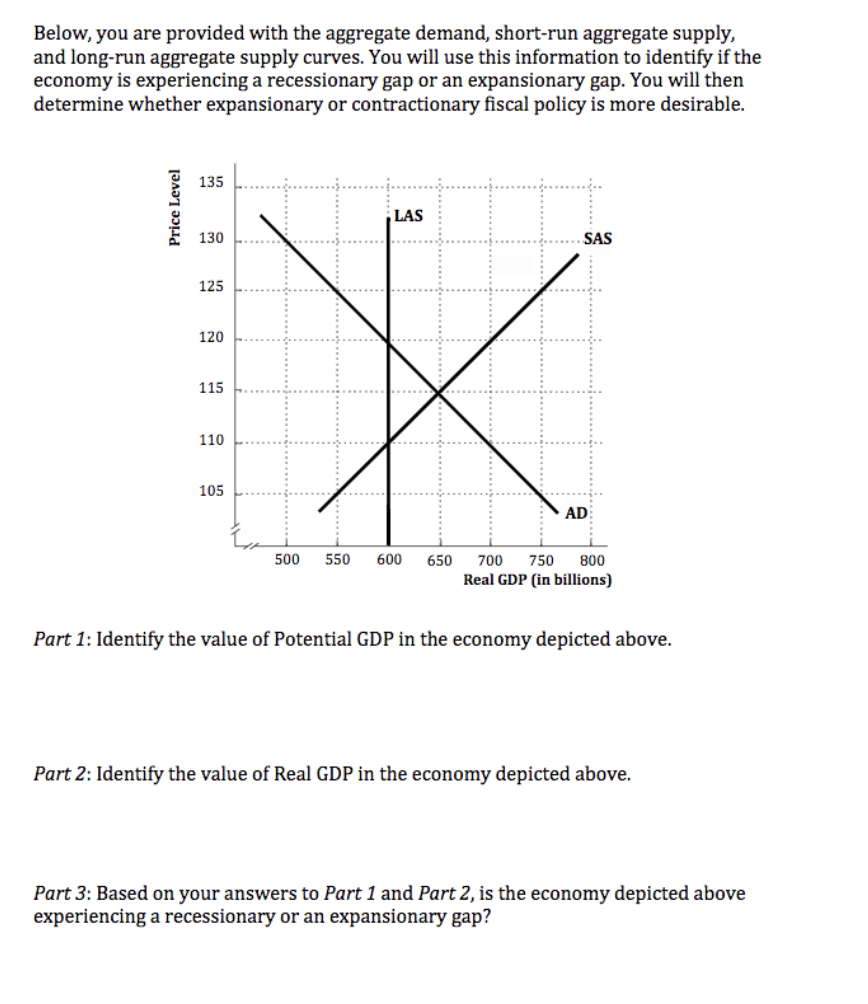Below, you are provided with the aggregate demand, short-run aggregate supply, and long-run aggregate supply curves. You will use this information to identify if the economy is experiencing a recessionary gap or an expansionary gap. You will then determine whether expansionary or contractionary fiscal policy is more desirable. 135 Price Level LAS 130 SAS 125 120 115 110 1 105 AD 500 550 600 650 700 750 800 Real GDP (in billions) Part 1: Identify the value of Potential GDP...

• ### Below, you are provided with the aggregate demand, short-run aggregate supply, and long-run aggregate supply curves....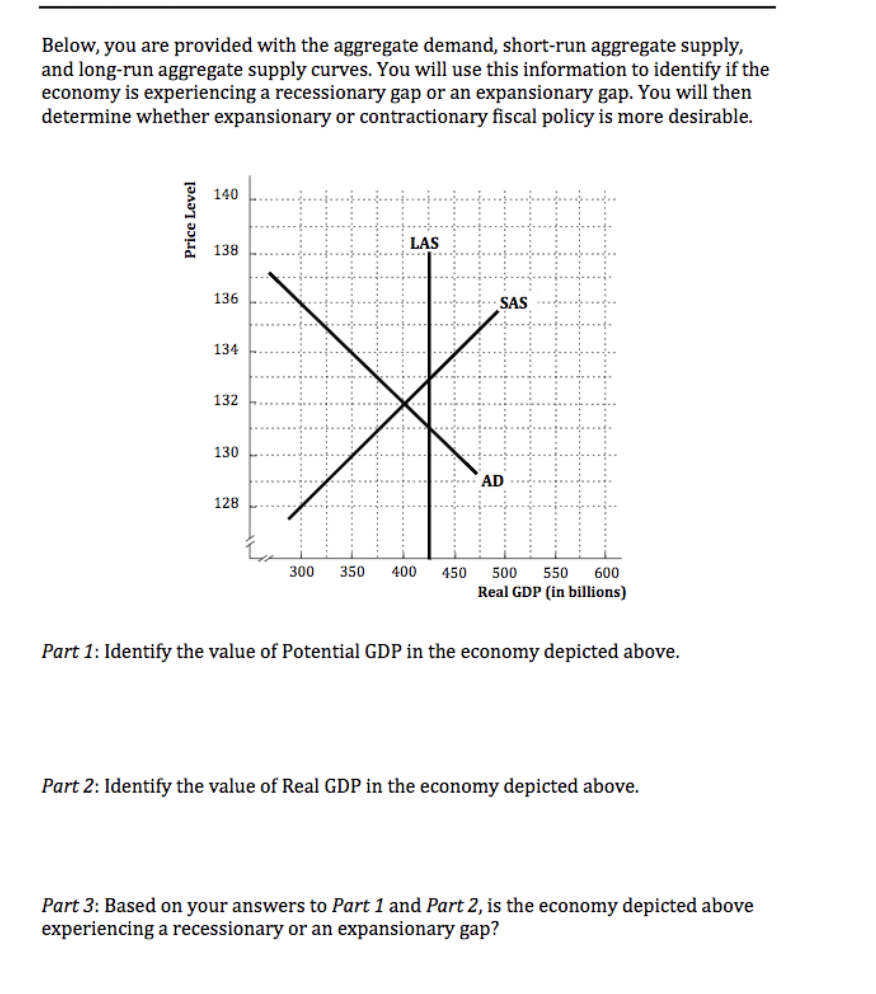Below, you are provided with the aggregate demand, short-run aggregate supply, and long-run aggregate supply curves. You will use this information to identify if the economy is experiencing a recessionary gap or an expansionary gap. You will then determine whether expansionary or contractionary fiscal policy is more desirable. 140 Price Level 138 LAS 136 SAS 134 X 132 130 AD 128 300 350 400 450 500 550 600 Real GDP (in billions) Part 1: Identify the value of Potential GDP...

• ### Short-run macroeconomic equilibrium occurs when: aggregate demand and short-run aggregate supply intersect. the equilibrium lies on...

Short-run macroeconomic equilibrium occurs when: aggregate demand and short-run aggregate supply intersect. the equilibrium lies on the long-run supply curve. the price level is constant in the short run. The two criteria – that aggregate demand and short-run aggregate supply intersect, and that the equilibrium lies on the long-run supply curve – must both be satisfied

• ### If the intersection of the short-run aggregate supply and aggregate demand curves is to the right...

If the intersection of the short-run aggregate supply and aggregate demand curves is to the right of the long-run aggregate supply curve, then the economy is Group of answer choices in a recession operating at more than full employment at full employment operating at less than full employment

• ### When the aggregate demand curve and the short-run aggregate supply curve intersect, a) the long-run aggregate...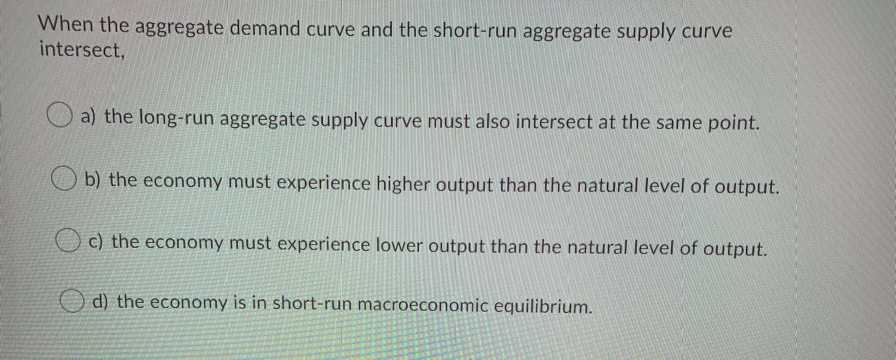When the aggregate demand curve and the short-run aggregate supply curve intersect, a) the long-run aggregate supply curve must also intersect at the same point. Ob) the economy must experience higher output than the natural level of output. o c) the economy must experience lower output than the natural level of output. o d) the economy is in short-run macroeconomic equilibrium. In a small economy in 2016, aggregate expenditure was \$900 million while GDP that year was \$750 million. Which...

• ### 2. Use the model of aggregate demand and short-run aggregate supply to explain how each of...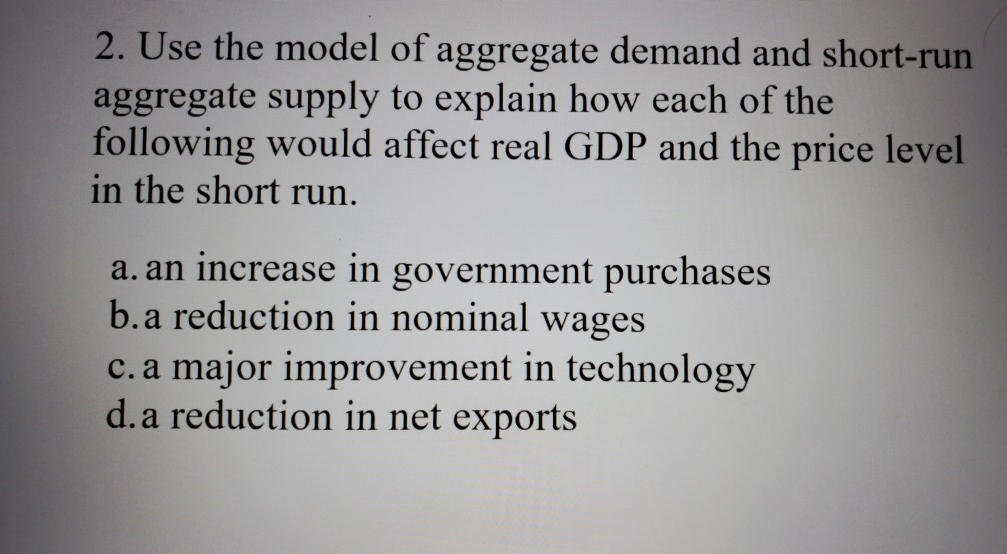2. Use the model of aggregate demand and short-run aggregate supply to explain how each of the following would affect real GDP and the price level in the short run. a. an increase in government purchases b.a reduction in nominal wages c. a major improvement in technology d. a reduction in net exports

• ### Using the IS-LM and Aggregate Supply-Aggregate Demand (AS-AD) models of Chapter 12 with a flat short-run...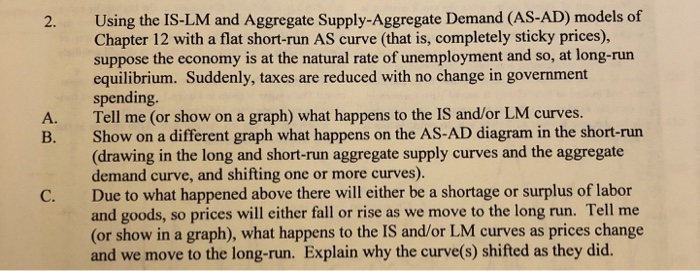Using the IS-LM and Aggregate Supply-Aggregate Demand (AS-AD) models of Chapter 12 with a flat short-run AS curve (that is, completely sticky prices), suppose the economy is at the natural rate of unemployment and so, at long-run equilibrium. Suddenly, taxes are reduced with no change in government spending. Tell me (or show on a graph) what happens to the IS and/or LM curves. Show on a different graph what happens on the AS-AD diagram in the short-run (drawing in the...